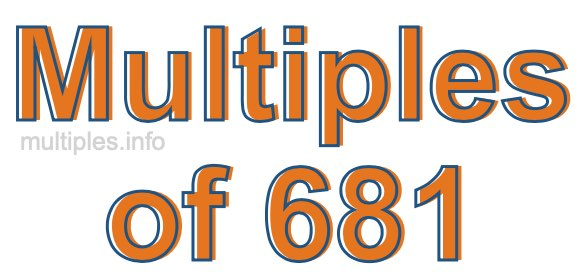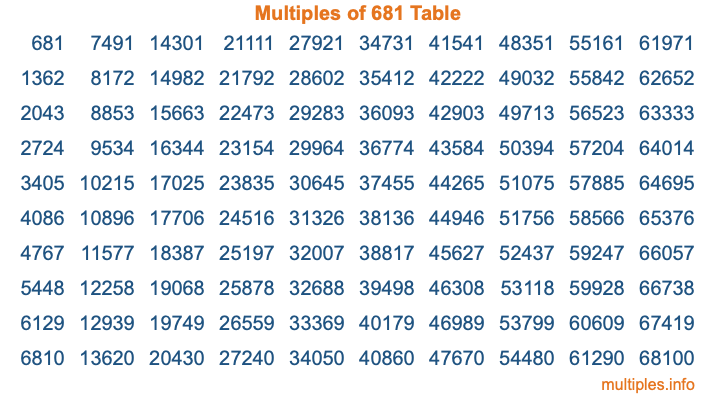Multiples of 681Welcome to the Multiples of 681 page. Here we will first teach you everything you will ever need to know about the multiples of 681, and then give you a study guide summary of everything we taught you to make sure you remember it all. Use this page to look up facts and learn information about the multiples of 681. This page will make you a multiples of six hundred eighty-one expert!

Definition of Multiples of 681
Multiples of 681 are all the numbers that when divided by 681 equal an integer. Each of the multiples of 681 are called a multiple. A multiple of 681 is created by multiplying 681 by an integer.

Therefore, to create a list of multiples of 681, you start with 1 multiplied by 681, then 2 multiplied by 681, then 3 multiplied by 681, and so on for as long as you want. Thus, the list of the first five multiples of 681 is 681, 1362, 2043, 2724, and 3405. To see a larger list of multiples of 681, see the printable image of Multiples of 681 further down on this page. We also have a category where you can choose any nth multiple of 681.

Multiples of 681 Checker
The Multiples of 681 Checker below checks to see if any number of your choice is a multiple of 681. In other words, it checks to see if there is any number (integer) that when multiplied by 681 will equal your number. To do that, we divide your number by 681. If the the quotient is an integer, then your number is a multiple of 681.

Is  a multiple of 681?

Least Common Multiple of 681 and ...
A Least Common Multiple (LCM) is the lowest multiple that two or more numbers have in common. This is also called the smallest common multiple or lowest common multiple and is useful to know when you are adding our subtracting fractions. Enter one or more numbers below (681 is already entered) to find the LCM.

Check out our LCM Calculator if you need more details about the Least Common Multiple or if you need the LCM for different numbers for adding and subtraction fractions.

nth Multiple of 681
As we stated above, 681 is the first multiple of 681, 1362 is the second multiple of 681, 2043 is the third multiple of 681, and so on. Enter a number below to find the nth multiple of 681.

th multiple of 681

Multiples of 681 vs Factors of 681
681 is a multiple of 681 and a factor of 681, but that is where the similarities end. All postive multiples of 681 are 681 or greater than 681. All positive factors of 681 are 681 or less than 681.

Below is the beginning list of multiples of 681 and the factors of 681 so you can compare:

Multiples of 681: 681, 1362, 2043, 2724, 3405, etc.

Factors of 681: 1, 3, 227, 681

As you can see, the multiples of 681 are all the numbers that you can divide by 681 to get a whole number. The factors of 681, on the other hand, are all the whole numbers that you can multiply by another whole number to get 681.

It's also interesting to note that if a number (x) is a factor of 681, then 681 will also be a multiple of that number (x).

Multiples of 681 vs Divisors of 681
The divisors of 681 are all the integers that 681 can be divided by evenly. Below is a list of the divisors of 681.

Divisors of 681: 1, 3, 227, 681

The interesting thing to note here is that if you take any multiple of 681 and divide it by a divisor of 681, you will see that the quotient is an integer.

Multiples of 681 Table
Below is an image of the first 100 multiples of 681 in a table. The table is in chronological order, column by column. The first column has the first ten multiples of 681, the second column has the next ten multiples of 681, and so on.The Multiples of 681 Table is also referred to as the 681 Times Table or Times Table of 681. You are welcome to print out our table for your studies.

Negative Multiples of 681
Although not often discussed or needed in math, it is worth mentioning that you can make a list of negative multiples of 681 by multiplying 681 by -1, then by -2, then by -3, and so on, to get the following list of negative multiples of 681:

-681, -1362, -2043, -2724, -3405, etc.

Multiples of 681 Summary
Below is a summary of important Multiples of 681 facts that we have discussed on this page. To retain the knowledge on this page, we recommend that you read through the summary and explain to yourself or a study partner why they hold true.

There are an infinite number of multiples of 681.

A multiple of 681 divided by 681 will equal a whole number.

681 divided by a factor of 681 equals a divisor of 681.

The nth multiple of 681 is n times 681.

The largest factor of 681 is equal to the first positive multiple of 681.

681 is a multiple of every factor of 681.

681 is a multiple of 681.

A multiple of 681 divided by a divisor of 681 equals an integer.

681 divided by a divisor of 681 equals a factor of 681.

Any integer times 681 will equal a multiple of 681.

Multiples of a Number
Here you can get the multiples of another number, all with the same attention to detail as we did for multiples of 681 on this page.

Multiples of
Multiples of 682
Did you find our page about multiples of six hundred eighty-one educational? Do you want more knowledge? Check out the multiples of the next number on our list!